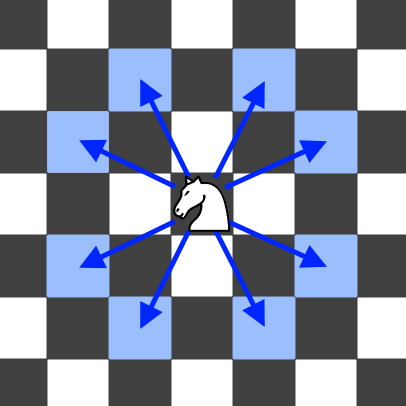# LeetCode: 688. “马”在棋盘上的概率¶

## 1、题目描述¶输入: 3, 2, 0, 0



• N 的取值范围为 [1, 25]
• K 的取值范围为 [0, 100]
• 开始时，“马” 总是位于棋盘上

## 2、解题思路¶

• DFS+记忆化

from functools import lru_cache

class Solution:
def knightProbability(self, N: int, K: int, r: int, c: int) -> float:
row, col = N, N

def get_surround(x, y):
surround = [(-2, -1), (-2, 1), (-1, -2), (1, -2), (2, -1), (2, 1), (-1, 2), (1, 2)]
for dx, dy in surround:
yield x + dx, y + dy

# 坐标验证
def available(m, n):
return 0 <= m < row and 0 <= n < col

@lru_cache(None)
def dfs(x, y, steps):
one_part = 1 / 8

if available(x, y):
if steps <= 0:
return 1
else:
ans = 0
for x1, y1 in get_surround(x, y):
ans += one_part * dfs(x1, y1, steps - 1)

return ans

else:
return 0

return dfs(r, c, K)